# CalcuDoku

## 7×7 Calcudoku+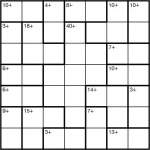Calcudoku+ 7×7

I had a request for a 7×7 Calcudoku-esque puzzle that uses only + operations, so I thought “why not?”.  And here it is.## Calcudoku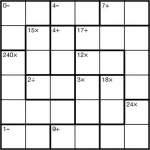Calcudoku 6×6 puzzle

It’s been an age since I’ve posted new puzzles on here directly, mainly because all my puzzle-making effort has been focused on Sudoku Xtra, Sudoku Pro, PuzzleMix and a major book I recently completed.

In any case, there’s no time like the present to fix that so here’s a simple 6×6 Calcudoku. Just place 1 to 6 in each row and column and make sure the given number results when the stated operator is applied between the numbers in each bold region.

## Samurai 3-grid Calcudoku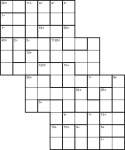Samurai 3-grid Calcudoku 6×6 puzzle

I’ve been posting a few new varieties of Sudoku puzzle in the daily puzzle section of PuzzleMix (i.e. the subscription section, which costs less than £1 a month for well over 1,000 puzzles a year). These aren’t especially unusual variations, but I’ve done a few different mixes (e.g. Sudoku-X 12×12 and Wraparound Sudoku 6×6), and I thought it was about time I posted a new mix here too.

So here’s a 3-grid Samurai Calcudoku puzzle.  You must place 1-6 in each of the 3 underlying 6×6 grids – I’m afraid these aren’t marked explicitly so you might want to draw in where the grid borders go so you don’t forget whilst solving.  Other than that, also place numbers so that the number given in each bold-lined cage results from applying the given operator between the numbers in that cage. Start with the highest number for division and subtraction. There is no restriction on repeating numbers in cages.

Good luck!## Crazy Calcudoku!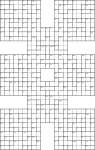Samurai 8-grid Calcudoku puzzle

Here’s probably the largest Calcudoku puzzle you’ve ever seen!  It’s made up of 8 underlying 9×9 grids, each of which must have 1 to 9 placed into each row and column, and then on top of this I’ve added the familiar Calcudoku regions.

For each Calcudoku region just place numbers such that the total after applying the stated operation between the numbers in the region is the one given.  For example, 7+ could be solved by 3 and 4 (3+4). For subtraction and division start with the largest number in the region, so 1- could also be solved with 3 and 4 (4-3).

Just to make it really clear, there are no 3×3 Sudoku box regions in this puzzle – just the 8 sets of rows and columns.

Good luck!## Inequality Calcudoku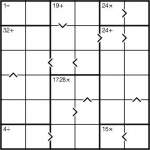Inequality Calcudoku puzzle

Here’s a fun little puzzle – a Calcudoku Inequality puzzle.

Just place 1 to 6 into each row and column whilst obeying the bold-lined cages’ operator totals. These give the result of applying the stated operator between all numbers in that region, so for example the result of adding together all the squares in a ‘24+’ region must be 24. Similarly the squares in the 1728x region must all multiply to that total. Unlike in Killer Sudoku, you can repeat a value within a cage (but you must still obey the constraint to not repeat a number in a row or column).

There are also some inequalities marked. These show that the value of one square is lower than the value of a square next to it. The arrow always points to the smaller number.

Good luck!## Toroidal Calcudoku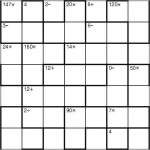Toroidal Calcudoku puzzle

Another easy Calcudoku in terms of its logic, although this one is a little trickier in practice since it’s 7×7 and is toroidal, meaning that some of the regions wrap around the edges of the grid and continue on the square directly opposite on that row/column.  Other than, just place 1 to 7 in each row and column whilst fulfilling the mathematical results given for each region. Unlike Killer Sudoku, you can repeat a digit within a cage (although of course not a row or column).

## Just a couple of Calcudoku

Today I was producing a few easy Calcudoku for a new book I’m working on, and had some left over so I thought I’d post them here. Nothing too special, but there’s a little bit of nice symmetry, which you don’t seem to generally find in published Calcudoku puzzles. (I don’t know why this is, since it only makes the puzzles better in my opinion!)

See older Calcudoku posts for the rules, if you need them (click the link in the side bar).

## Consecutive Samurai CalcuDoku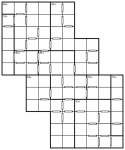Consecutive Samurai CalcuDoku puzzle

Now this is an interesting puzzle!  At first glance it looks like it can’t possibly have a unique solution, since there are no givens and only 9 cages on the entire 3-grid Samurai puzzle (made up of 6×6 grids).  But in fact it uses only simple logic to solve, and it unravels pretty quickly once you get going.  It’s a pretty powerful demonstration of how much you can strip a puzzle back and still keep it entirely reasonable in terms of difficulty.  In fact it’s arguably too easy…

The rules are what you’d expect if you’ve been following previous puzzles, but in summary you must: (deep breath!)

• Place 1 to 6 into each row and column of the three underlying 6×6 grids
• Place numbers into each of the bold-lined cages so that they add up to the number at the top-left (or in the case of the 40x cage, multiply up to that value)
• Wherever a white bar divides two squares, the numbers in those two squares must be consecutive (so they must be one of these pairs: 1&2, 2&3, 3&4, 4&5 or 5&6)
• Where no white bar divides two squares, the numbers are non-consecutive

Good luck!

## Samurai 5-grid Killer CalcuDoku puzzle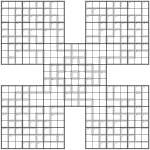Samurai 5-grid Killer Calcudoku puzzle

Here’s an interesting puzzle.  It’s a 5-grid Samurai Killer CalcuDoku, which means that it has the 3×3 boxes from Killer Sudoku but otherwise works like a CalcuDoku puzzle, albeit a 5-grid Samurai one!  All of the operations in this puzzle are addition, so aren’t shown.

Can you place 1 to 9 into each row, column and 3×3 box of each of the underlying 9×9 Sudoku grids, whilst also placing numbers so that each inner cage adds up to the total given at its top-left corner?  Numbers can be repeated within these cages (pretty obviously, given how large some of them are!).

There are quite a lot of single digit cells, suggesting (truthfully) that this isn’t actually a very difficult puzzle – but it’s a good proof of concept, I think.  You can create really huge cages if you want, without making the puzzle difficult (of course, the easiest way to solve these is to essentially ignore the cage completely, or at least until it is nearly finished).  This particular puzzle does not require you to do any complex maths at all.

Good luck!

## As Easy as 11, 22, 33… a Killer CalcuDoku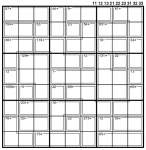As easy as 11, 22, 33 Killer CalcuDoku

Here’s a relaxing puzzle for a Sunday… or maybe not!  Can you complete this Killer CalcuDoku puzzle made up of 1s, 2s and 3s?

Place 11, 12, 13, 21, 22, 23, 31, 32 or 33 into each square so that the result of applying the specified operation to each cage is the given number.  (Start with the highest number in the cage for subtraction and division cages).  Also, can you obey the standard Sudoku constraints: place each of the 9 different numbers once per row, column and bold-lined 3×3 box?  You can repeat numbers within a cage, however, if you wish (which is why it’s a Killer CalcuDoku, not a Killer Sudoku Pro, in my terminology!  It’s also why it has solid cages, rather than dashed-line cages).

The logic isn’t too tricky, but for speed you might find a calculator helps you make a few of the logically easy deductions…Good luck!